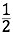# Calling to Order

## OBJECTIVE: To learn about order relationships between real numbers

In this lesson, you'll learn about order relationships between real numbers and how they are depicted on the real number line. You'll also learn how to compare numbers based on their positions in the number line. The set of real numbers has order. ﻿In the real number line, numbers get smaller as you go to the left and larger as you go to the right.

## TRICHOTOMY PROPERTY

If x and y are real numbers, only one of the following statements is true: x < y          x = y          x > y In words, either x is less than y, x is equal to y, or x is greater than y. This relationship is known as the Trichotomy Property. A number is greater than ( > ) any number to its left on the number line. Examples: In the real number line below, 8 > 3, 3 > -1, and -1 > -4. A number is less than ( < ) any number to its right on the number line. Examples: In the real number line below, -4 < -1.5, -1.5 < 0, 0 <Remember: The arrowhead always points to the smaller number.
Use the applet below to practice comparing real numbers. Repeat the exercise as often as needed to master the concept.

## In this lesson, you learned about order relationships between real numbers and how to compare them based on their positions in the real number line.

In future lessons, you'll learn how to graph subsets of real numbers on the real number line. Did you ENJOY today's lesson?